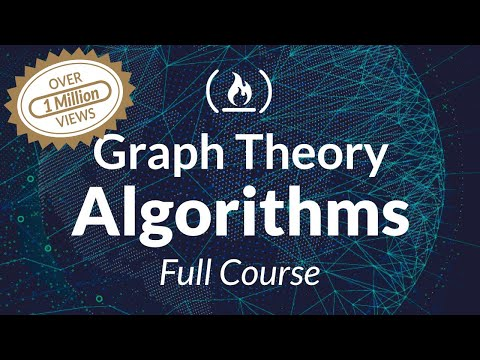# Algorithms Course – Graph Theory Tutorial from a Google Engineer## Algorithms Course – Graph Theory Tutorial from a Google Engineer1435827 People Read – 39592 People Liked – You Can Also Like

This full course provides a complete introduction to Graph Theory algorithms in computer science. Knowledge of how to create and design excellent algorithms is an essential skill required in becoming a great programmer.

You will learn how many important algorithms work. The algorithms are accompanied by working source code in Java to solidify your understanding.

💻 Code: https://github.com/williamfiset/algorithms
🔗 Slides: https://github.com/williamfiset/Algorithms/tree/master/slides/graphtheory

⭐️ Course Contents ⭐️
⌨️ (0:00:00) Graph Theory Introduction
⌨️ (0:13:53) Problems in Graph Theory
⌨️ (0:23:15) Depth First Search Algorithm
⌨️ (0:33:18) Breadth First Search Algorithm
⌨️ (0:40:27) Breadth First Search grid shortest path
⌨️ (0:56:23) Topological Sort Algorithm
⌨️ (1:09:52) Shortest/Longest path on a Directed Acyclic Graph (DAG)
⌨️ (1:19:34) Dijkstra’s Shortest Path Algorithm
⌨️ (1:43:17) Dijkstra’s Shortest Path Algorithm | Source Code
⌨️ (1:50:47) Bellman Ford Algorithm
⌨️ (2:05:34) Floyd Warshall All Pairs Shortest Path Algorithm
⌨️ (2:20:54) Floyd Warshall All Pairs Shortest Path Algorithm | Source Code
⌨️ (2:29:19) Bridges and Articulation points Algorithm
⌨️ (2:49:01) Bridges and Articulation points source code
⌨️ (2:57:32) Tarjans Strongly Connected Components algorithm
⌨️ (3:13:56) Tarjans Strongly Connected Components algorithm source code
⌨️ (3:20:12) Travelling Salesman Problem | Dynamic Programming
⌨️ (3:39:59) Travelling Salesman Problem source code | Dynamic Programming
⌨️ (3:52:27) Existence of Eulerian Paths and Circuits
⌨️ (4:01:19) Eulerian Path Algorithm
⌨️ (4:15:47) Eulerian Path Algorithm | Source Code
⌨️ (4:23:00) Prim’s Minimum Spanning Tree Algorithm
⌨️ (4:37:05) Eager Prim’s Minimum Spanning Tree Algorithm
⌨️ (4:50:38) Eager Prim’s Minimum Spanning Tree Algorithm | Source Code
⌨️ (4:58:30) Max Flow Ford Fulkerson | Network Flow
⌨️ (5:11:01) Max Flow Ford Fulkerson | Source Code
⌨️ (5:27:25) Unweighted Bipartite Matching | Network Flow
⌨️ (5:38:11) Mice and Owls problem | Network Flow
⌨️ (5:46:11) Elementary Math problem | Network Flow
⌨️ (5:56:19) Edmonds Karp Algorithm | Network Flow
⌨️ (6:05:18) Edmonds Karp Algorithm | Source Code
⌨️ (6:10:08) Capacity Scaling | Network Flow
⌨️ (6:19:34) Capacity Scaling | Network Flow | Source Code
⌨️ (6:25:04) Dinic’s Algorithm | Network Flow
⌨️ (6:36:09) Dinic’s Algorithm | Network Flow | Source Code

Read hundreds of articles on programming: https://www.freecodecamp.org/news